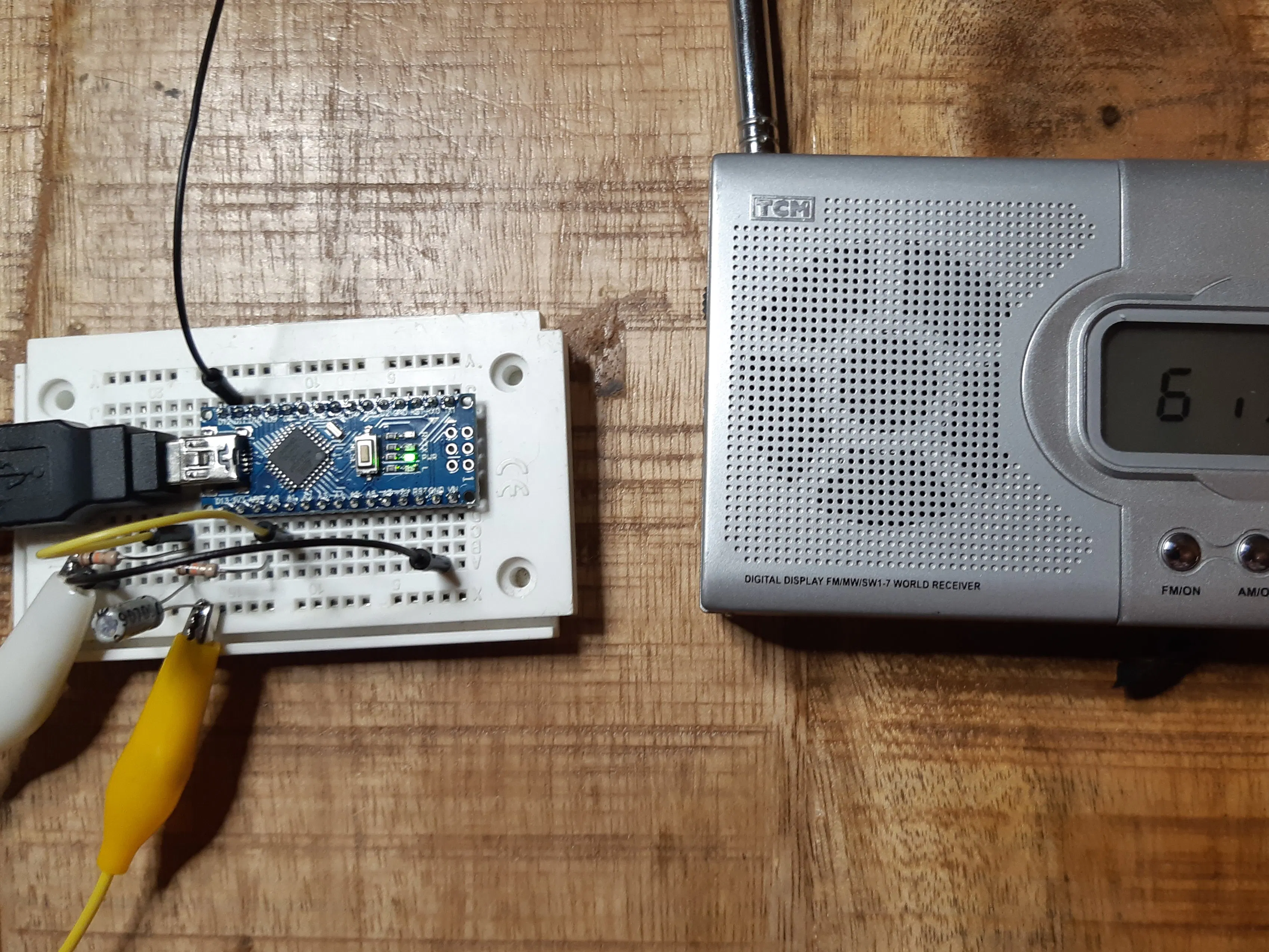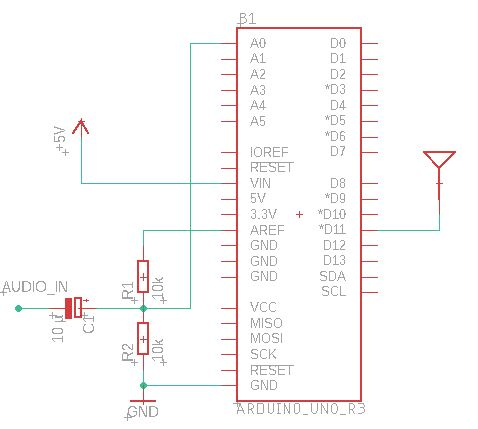Project tutorial# (Ab)use an Arduino as AM Music Transmitter! © CC BY-SA

See how an Arduino can be transmit music over AM with only a few lines of code.

• 9,961 views
• 41 respects

## Apps and online services

Cool things can be done with ATmega MCUs. So I got the idea to build an AM transmitter with an Arduino Nano (any other Arduino is also possible)

The question is, how can amplitude modulation (AM) be achieved on a digital device? AM means that the amplitude of a carrier frequency is changed according to the amplitude of the input signal which we want to transmit. Mathematically spoken, we multiply the carrier with the input signal. But as an ATmega has only digital outputs, it can give only two values: high and low. Frankly spoken, there is no way to get real AM out of an Arduino.

Nevertheless it is possible to produce some output signal that can be heard clearly with an ordinary AM radio receiver!

The solution consists of transforming the analog input signal into a PWM signal, using the ADC and the 16-bit timer/counter1 of the ATmega MCU.

The timer/counter2 generates the carrier signal, which can be varied in a wide range between 31KHz and 8MHz.

This carrier signal is output on pin D11 in the rhythm of the PWM signal. The AM radio behaves like a low pass filter on the PWM, so we get back the original audio signal there.

This is only a proof of concept, so I kept it simple. If you really want to use this as RF transmitter, you should add a low-pass fillter for anti-aliasing to the audio input and a low pass against the harmonics to the RF output.

## Code

##### Arduino AM TransmitterC/C++
```/*This work is licensed under the Creative Commons Attribution-ShareAlike 4.0 International License. To view a copy of this license, visit http://creativecommons.org/licenses/by-sa/4.0/.*/

#define ANTENNA_PIN PB3 //Arduino Nano/Uno D11

// the setup function runs once when you press reset or power the board
void setup() {
Serial.begin(115200);

//Carrier Frequency generation
uint32_t fTransmit = 600; //KHz
DDRB |= (1 << ANTENNA_PIN);
TCCR2A = (0 << COM2A1) + (1 << COM2A0); //Toggle OC0A on Compare Match
TCCR2A |= (1 << WGM21) + (0 << WGM20); //CTC
TCCR2B = (0 << CS22) + (0 << CS21) + (1 << CS20); //No Prescaling
OCR2A = F_CPU / (2000 * fTransmit) - 1;

char strbuf;
sprintf(strbuf, "Will broadcast at %d KHz", (F_CPU / (2 * (1 + OCR2A)) / 1000));
Serial.println(strbuf);

//PWM Signal generation
//TCCR1A |= (0 << WGM11) + (1 << WGM10);  //Fast PWM 8 Bit
//TCCR1A |= (1 << WGM11) + (0 << WGM10);  //Fast PWM 9 Bit
TCCR1A |= (1 << WGM11) + (1 << WGM10); //Fast PWM 10 Bit
TCCR1B = (1 << WGM12);
TCCR1B |= (0 << CS12) + (0 << CS11) + (1 << CS10); //No Prescaling
TIMSK1 = (1 << OCIE1A) + (1 << TOIE1);

ADMUX = (1 << REFS1) + (1 << REFS0); //Reference internal 1.1V
}

ISR(TIMER1_OVF_vect) {
DDRB |= (1 << ANTENNA_PIN);
}

ISR(TIMER1_COMPA_vect) {
DDRB &= ~(1 << ANTENNA_PIN);
}

void loop() {
}
```

## Schematics#### Out of My League - Music on Arduino

Project tutorial by MrZmann

• 1,418 views
• 4 respects

#### Music Reactive LED Strip

Project showcase by buzzandy

• 78,012 views
• 154 respects

#### TuneGlass: Can We Make MUSIC Using Our EYES???

Project tutorial by ashraf_minhaj

• 1,963 views
• 13 respects

#### Making Arduino-Based RC Transmitter of USB Flight Simulator

Project showcase by tsar_

• 1,144 views
• 6 respects

#### Add WiFi to Arduino UNO

• 298,435 views